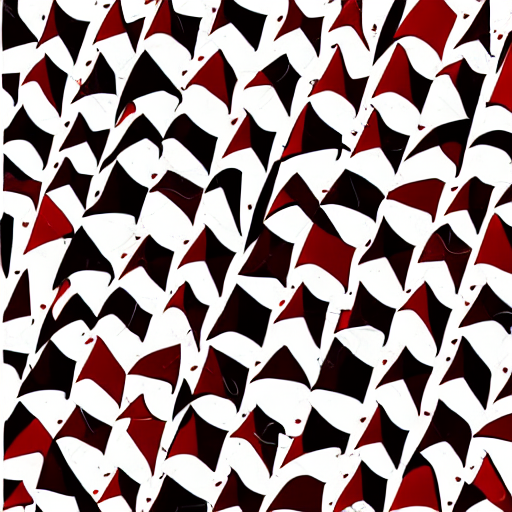Perl is overpowered and can replace grep,sed,awk,cut, etc

I spent all day today learning perl and perl regexes (PRCE's). With this wonderful oneliner as the climax

perl -0777 -pe 's/Exercise \d\.\d\.\d\d?\.(.*?)(?=^Exercise|\z)/\\begin{exercise}\n$1\\end{exercise}\n/smg' What does it do? it converts text like this Exercise 1.2.3. Suppose$V$is a finite dimensional vector space and$F$is a field ... Exercise 1.2.4. Let$F$denote the set of fat people and$M$denote your mom. Prove that$F \cap M \ne \emptyset$. Into this \begin{exercise} Suppose$V$is a finite dimensional vector space and$F$is a field ... \end{exercise} \begin{exercise} Let$F$denote the set of fat people and$M$denote your mom. Prove that$F \cap M \ne \emptyset$. \end{exercise} This is useful for getting mathpix output into the right form for my understanding-analysis-solutions project. How does it do it? well first the commandline flags (see man perlrun) • -0777 makes perl slurp files whole • -p wraps the program in a while-print-loop Then the regex (see man perlre). I've added the x option so it works with comments s/ # match "Exercise 1.2.3." (notice the ending dot) Exercise \d\.\d\.\d\d?\. # match the body, the ? makes it lazy so when the next # pattern matches it'll exit (.*?) # a zero width lookahead patern which matches ^Exercise # or end of file (\z). "zero width" means it won't be # included in the match. it only "looks ahead" (?=^Exercise|\z) # Time to replace / # Replace it with this,$1 refers to the first capture group
\\begin{exercise}\n$1\\end{exercise}\n # the regex options # s -> makes . match newlines # m -> makes ^,$ work in multiline regexes
# g -> global, replace all occurrences
/smgx
Want to learn this arcane power? spend a few hours reading the perl manpages. Specifically read perlre and perlrun.
I'm convinced that grep, awk, sed etc are bloat! perl can do everything they do better (sometimes in slightly more code). How many times have I struggled with grep not being powerful enough! Never again. Perl is the new sed 11q